# Remove/Add Back Point to the Calculations HelpWe will use the following example to see how to remove a point from the calculations or add it back to the calculations. Suppose you have made an X chart using the following data: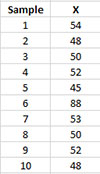The X chart from these data are shown below. The average = 54 with an upper control limit (UCL) = 84.7 and a lower control limit (LCL) = 23.3. There is one point above the UCL. You know the reason why that point is out of control and you want to remove it from the calculations.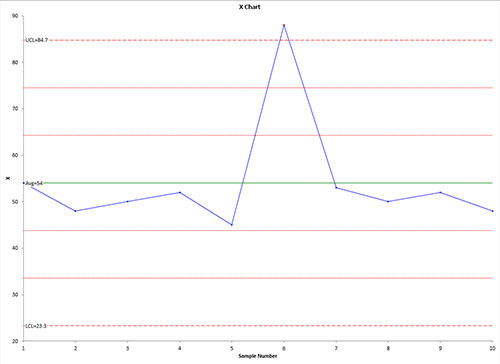To use the remove a point (or add it back) to the calculations, you must select a point on the control chart before you select “Actions” from the “Control Charts” panel on the SPC for Excel ribbon. This is a two-step process in Excel. First select the series containing the results (e.g., subgroup averages). The entire series is selected. Then select the point where you want to add/remove the comment. See Split Control Limits if you need more information on how to select the point on the chart.

Once the single point is selected, select “Actions” in the “Control Charts” panel on the SPC for Excel ribbon.

Select the “Remove from/Add back this point to the calculations” from the Control Chart Actions form.

The point is then removed from the calculations if it is present or added back in if it is not present in the calculations. In this case, it is removed and the chart now looks like: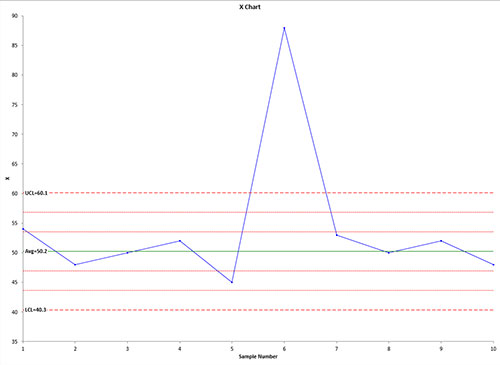The new average = 50.2 with an UCL = 60.1 and an LCL = 40.3.

You can tell from the chart if a point has been deleted from the calculations. It will have a border only but no fill as shown below: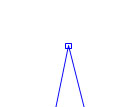Removing a point from the calculations does change the worksheet containing the data. The value that has been deleted has been shaded: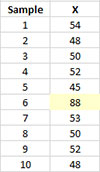To add a point back to the chart, repeat the above process. The shading on the worksheet will be removed when the point is added back to the calculations.